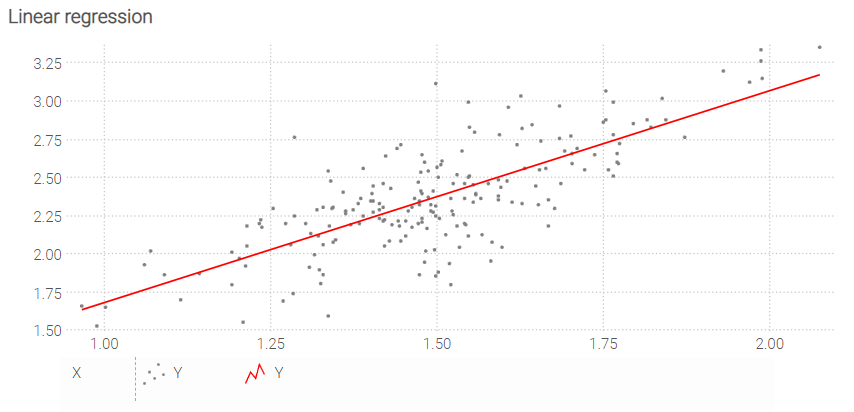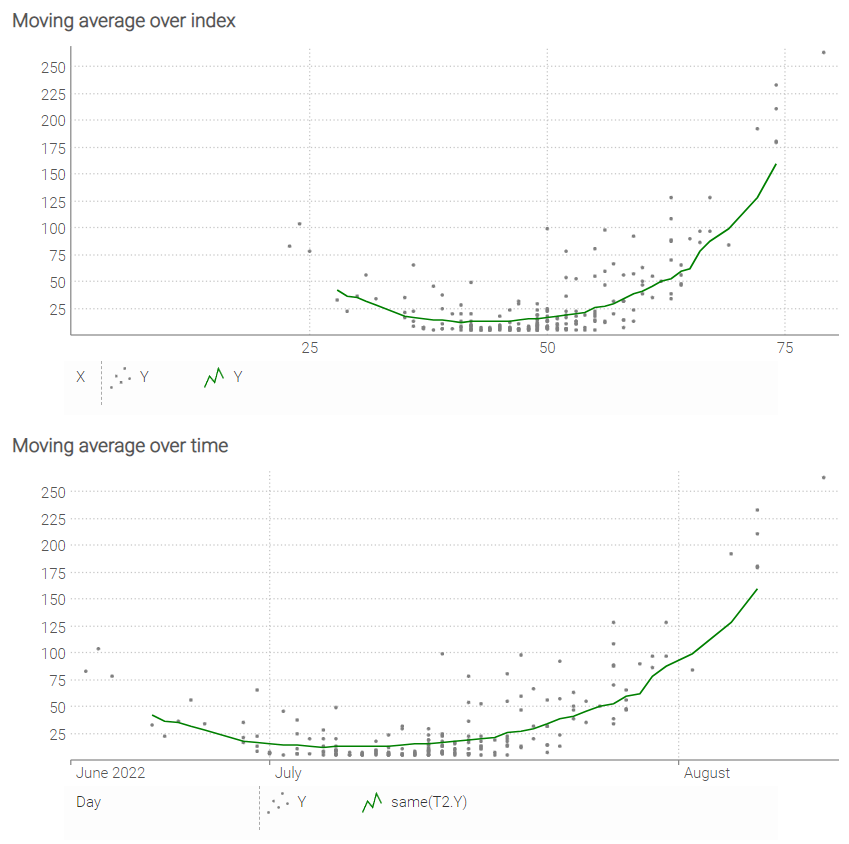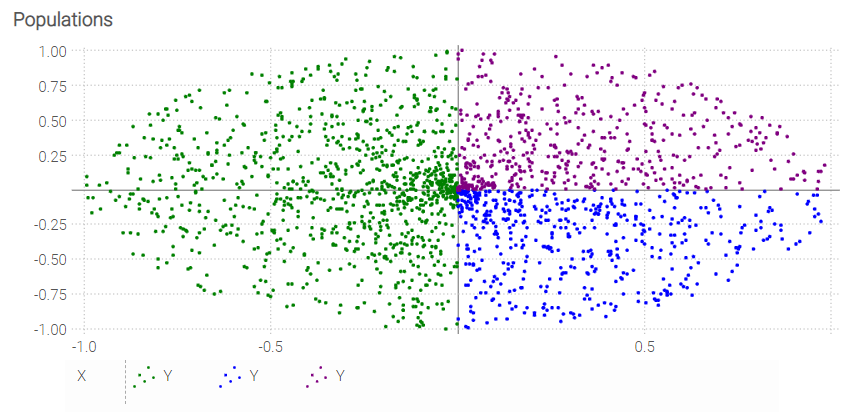# chart

## chart, tile type

The chart supports composing a series of internal tiles. Unlike the other tiles, the chart tile can take data from multiple tables as input.

### Illustration: linear regressionN = 200
table T = extend.range(N)

meanX = 1.5
sigmaX = 0.2
T.X = random.normal(meanX into T, sigmaX)

slope = 1.3
meanB = 0.4
sigmaB = 0.2
T.Y = slope * T.X + random.normal(meanB into T, sigmaB)

regressionA = (N * sum(T.X * T.Y) - sum(T.X) * sum(T.Y)) / (N * sum(T.X^2) - sum(T.X)^2)
regressionB = (sum(T.Y) - sum(T.X) * regressionA) / N

table LinReg = with
[| as X, as Y |]
[| min(T.X) into Scalar, (min(T.X) into Scalar) * regressionA + regressionB |]
[| max(T.X) into Scalar, (max(T.X) into Scalar) * regressionA + regressionB |]

show chart "Linear regression" a1d4 { precision: 5 ; axisMin: min } with
scatter T.X
T.Y { color: gray }
plotxy LinReg.X
LinReg.Y { color: red }
order by LinReg.X


### Illustration: moving averageN = 200
table T = extend.range(N)

meanX = 50
sigmaX = 10
T.X = round(random.normal(meanX into T, sigmaX))

slope = 0.4
meanB = -18
sigmaB = 2
T.Y = 5 + (slope * T.X + random.normal(meanB into T, sigmaB)) ^ 2

table T2[X] = by T.X
T2.Y = avg(T.Y) over X = [-8..8]

minX = min(T.X)
maxX = max(T.X)

show chart "Moving average over index" a1d4 with
haxis by T2.X
scatter T.X
T.Y { color: gray }
where T2.X > minX + 4 and T2.X < maxX - 4
plot by T2.X
T2.Y { color: green }

T.Day = date(2022,05,25) + T.X
T2.Day = date(2022,05,25) + T2.X

show chart "Moving average over time" a5d8 with
haxis by T2.Day
scatter T.Day
T.Y { color: gray }
where T2.X > minX + 4 and T2.X < maxX - 4
plot by T2.Day
same(T2.Y) { color: green }


### Illustration: populationsN = 2000
table T = extend.range(N)

const PI = 3.14159274101
T.Theta = random.uniform(0,2 * PI into T)
T.R = random.uniform(0 into T, 1)
T.X = T.R * cos(T.Theta)
T.Y = T.R * sin(T.Theta)

show chart "Populations" a1d4 with
where T.X < 0
scatter T.X
T.Y { color: green }
where T.X >= 0
where T.Y < 0
scatter T.X
T.Y { color: blue }
where T.Y >= 0
scatter T.X
T.Y { color: purple }

User Contributed Notes
0 notes + add a note